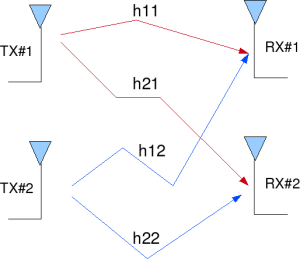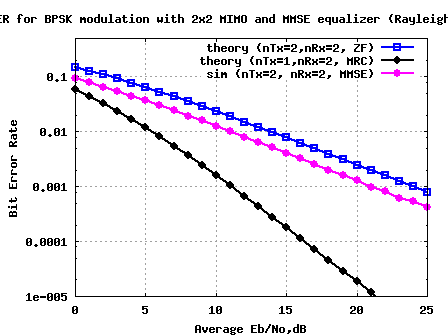- DSP log - http://www.dsplog.com -

MIMO with MMSE equalizer

Posted By Krishna Sankar On November 2, 2008 @ 8:35 pm In MIMO | 245 Comments

In a previous post, we had discussed a 2×2 MIMO transmission using BPSK modulation in Rayleigh channel with a Zero Forcing equalizer . The simulated results with the 2×2 MIMO system  with zero forcing equalizer showed matching results as obtained in for a 1×1 system for BPSK modulation in Rayleigh channel . In this post, we will discuss a different equalization approach called Minimum Mean Square Error (MMSE) equalization. We will assume that the channel is a flat fading Rayleigh multipath channel  and the modulation is BPSK.

The background material on the MIMO channel has been described in the post on Zero Forcing equalizer . The text is repeated again for easy readability.

## 2×2 MIMO channel

In a 2×2 MIMO channel, probable usage of the available 2 transmit antennas can be as follows:

1. Consider that we have a transmission sequence, for example

2. In normal transmission, we will be sending in the first time slot, in the second time slot, and so on.

3. However, as we now have 2 transmit antennas, we may group the symbols into groups of two. In the first time slot, send and from the first and second antenna. In second time slot, send and from the first and second antenna, send and in the third time slot and so on.

4. Notice that as we are grouping two symbols and sending them in one time slot, we need only time slots to complete the transmission – data rate is doubled !5. This forms the simple explanation of a probable MIMO transmission scheme with 2 transmit antennas and 2 receive antennas.Figure: 2 Transmit 2 Receive (2×2) MIMO channel

## Other Assumptions

1. The channel is flat fading – In simple terms, it means that the multipath channel has only one tap. So, the convolution operation reduces to a simple multiplication. For a more rigorous discussion on flat fading and frequency selective fading, may I urge you to review Chapter 15.3 Signal Time-Spreading from [DIGITAL COMMUNICATIONS: SKLAR]2. The channel experience by each transmit antenna is independent from the channel experienced by other transmit antennas.

3. For the transmit antenna to receive antenna, each transmitted symbol gets multiplied by a randomly varying complex number . As the channel under consideration is a Rayleigh channel, the real and imaginary parts of are Gaussian distributed having mean and variance .

4. The channel experienced between each transmit to the receive antenna is independent and randomly varying in time.

5. On the receive antenna, the noise has the Gaussian probability density function with

with and .

7. The channel is known at the receiver.

## Minimum Mean Square Error (MMSE) equalizer for 2×2 MIMO channel

Let us now try to understand the math for extracting the two symbols which interfered with each other. In the first time slot, the received signal on the first receive antenna is,

.

.

where

, are the received symbol on the first and second antenna respectively,

is the channel from transmit antenna to receive antenna,

is the channel from transmit antenna to receive antenna,

is the channel from transmit antenna to receive antenna,

is the channel from transmit antenna to receive antenna,

, are the transmitted symbols and

is the noise on receive antennas.

We assume that the receiver knows , , and . The receiver also knows and . For convenience, the above equation can be represented in matrix notation as follows:

.

Equivalently,

The Minimum Mean Square Error (MMSE) approach tries to find a coefficient which minimizes the criterion,

.

Solving,

.

When comparing to the equation in Zero Forcing equalizer , apart from the term both the equations are comparable. Infact, when the noise term is zero, the MMSE equalizer reduces to Zero Forcing equalizer .

## Simulation Model

The Matlab/Octave script performs the following

(a) Generate random binary sequence of +1′s and -1′s.

(b) Group them into pair of two symbols and send two symbols in one time slot

(c) Multiply the symbols with the channel and then add white Gaussian noise.

(e) Perform hard decision decoding and count the bit errors

(f) Repeat for multiple values of and plot the simulation and theoretical results.Figure: BER plot for 2×2 MIMO with MMSE equalization for BPSK in Rayleigh channel

## Summary

Compared to the Zero Forcing equalizer case, at BER point, it can be seen that the Minimum Mean Square Error (MMSE) equalizer results in around 3dB of improvement.## References

[DIG-COMM-BARRY-LEE-MESSERSCHMITT] Digital Communication: Third Edition, by John R. Barry, Edward A. Lee, David G. Messerschmitt[WIRELESS-TSE, VISWANATH]Fundamentals of Wireless Communication, David Tse, Pramod ViswanathURL to article: http://www.dsplog.com/2008/11/02/mimo-mmse-equalizer/

URLs in this post:

 Zero Forcing equalizer: http://www.dsplog.com/2008/10/24/mimo-zero-forcing/

 BPSK modulation in Rayleigh channel: http://www.dsplog.com/2008/08/10/ber-bpsk-rayleigh-channel/

 Rayleigh multipath channel: http://www.dsplog.com/2008/07/14/rayleigh-multipath-channel/